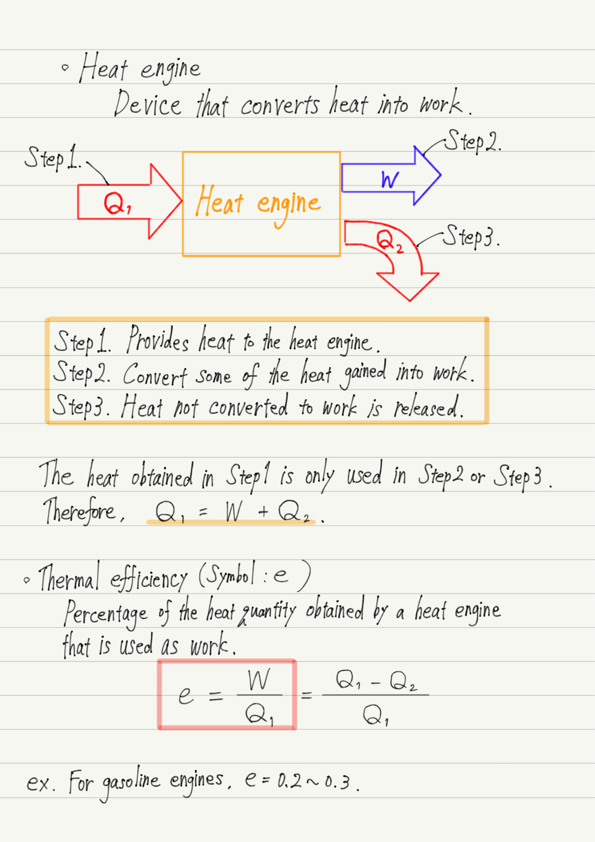# Thermal Efficiency

If I asked you to give an example of how thermodynamics has helped you in your life, what would you think of?

The first thing that comes to mind is undoubtedly the engine.
Gasoline engines in automobiles, diesel engines in ships, and steam engines in locomotives are all devices that convert heat generated by burning fuel into work.
This conversion of thermal energy into work is called a heat engine.

## Function of heat engine

The specific structure of an engine is not the subject here.
What we are interested in as physics is not “how the machine works” when a heat engine converts heat into work, but “what happens as a phenomenon”.
Therefore, in the following, we will ignore the specific engine structure.

First, heat is naturally required to operate a heat engine.
In the case of a car, heat is obtained by burning gasoline.
The heat is then converted to work, but not all of the heat gained is converted to work.
Heat that does not do any work is released as waste heat.

In summary, the function of a heat engine is as follows.

Step1. Provides heat to the heat engine.
Step2. Convert some of the heat gained into work.
Step3. Heat not converted to work is released.

To give a specific numerical example, if 1000 J of heat is obtained in Step1 and 300 J of work is done in Step2, the amount of heat released in Step3 is 700 J.
Since energy does not increase or decrease along the way, the sum of Steps 2 and 3 will always equal the amount obtained in Step 1!

## Efficiency of heat engine

We have explained that not all of the heat gained by a heat engine is converted to work, but what percentage is actually converted to work?

The percentage of heat received by the heat engine that is converted into work is called the thermal efficiency. (the formula is presented in the summary at the end.)
The higher the thermal efficiency, the more efficient the heat engine. By the way, the thermal efficiency of a steam engine is about 0.1 to 0.2 (ratio of the heat quantity obtained to 1).

So what do you think the thermal efficiency of gasoline engines is?

Is it 0.5?　Would it be 0.7?　Or almost 1?

The thermal efficiency is not much different than a steam engine.
This means that 70-80% of the gasoline you put in isn’t used to power it!

We can see that it is very difficult to improve thermal efficiency.
I will not go into the depth of this issue as it is in the field of mechanical engineering.
If you are interested, please check it out by yourself!

## Summary of this lecture## Next Time

Learn about the second law of thermodynamics!

https://www.yukimura-physics.com/entry/therm-f10

error: Content is protected !!